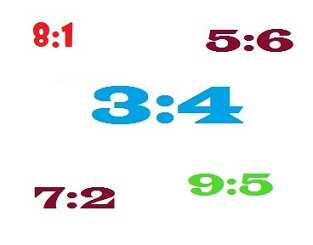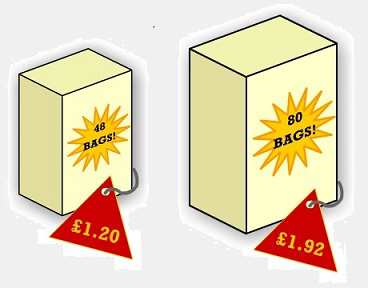# Ratio

### What is a ratio?

A ratio is a comparison between two or more values, usually written with a colon between the values. For example, we would read the ratio 3:4 as ‘three to four’.

###Suppose a certain shade of grey paint is made by mixing 3 parts black paint with 4 parts white paint, then the black and white paint are mixed in the ratio 3:4. If we were asked what the ratio of white to black paint was, we would have to say 4:3. So it is important that we get our ratio the right way round.

### Comparing ratios

To make a comparison between information given in the form of ratios, we can calculate unit amounts, or in other words, find out what one part is worth.

####My favourite teabags come in boxes of 48 or 80. The box containing 48 teabags costs £1.20 whilst the box containing 80 teabags costs £1.92. Which box is better value?

#### Solution

How do we compare prices?  We find the cost (pence) per teabag for each box.

Small box: 120p ÷ 48 = 2.5p per teabag

Large box: 192p ÷  80 = 2.4p per teabag

The cost per teabag is less for the larger box, so this is better value.

### Simplifying ratios

To simplify a ratio, divide through each term by a number which divides into both.

#### Example 2

Simplify the ratio 9:12.

#### Solution

Both numbers can be divided by three, so the ratio could be written as 3:4.

### Increasing or decreasing in a ratio

Sometimes we may want to increase or decrease a quantity according to a ratio. To do this
1. divide - to calculate what one part is worth
2. multiply - to calculate the new quantity

#### Example 3

I am going to be cooking dinner for 6 people. The recipe I am following suggests that I use 200g of rice for 4 people. How much rice should I use for 6?

#### Solution

Amount of rice required per person:  200g ÷ 4 = 50g per person

So for 6 people, I will require:   6 × 50g = 300g

### Sharing a quantity in a ratio

Sometimes we may want to share out a quantity in a ratio. To do this

1. add up the total number of parts
2. calculate what one part is worth
3. calculate what each person will receive
4. check your calculation is correct by making sure all the parts add up to the total

#### Example 4

In his will Uncle Joe has left his savings of £10,000 to be split between his daughter and grandson in the ratio 7:3. How much money will each receive?

#### Solution

1. In total we have 7 + 3 = 10 parts
2. So one part is worth £10,000 ÷ 10 = £1,000
3. Uncle Joe’s daughter will receive 7 × £1,000 = £7,000.  Uncle Joe’s grandson will receive 3 × £1,000 = £3,000.
4. Check that the money the daughter and grandson receive adds up to total amount left by Uncle Joe. £7,000 + £3,000 = £10,000

### Exercise 1

In a bag I have 8 black balls and 3 red. What is the ratio of red to black balls?

#### Solution

We have 3 red balls to 8 black balls, so the ratio is 3:8.

### Exercise 2

Which of the following bottles of water is the cheapest?

Welsh Wizard Irish Mist Eau Francais Scotch Broth
£1.62 for 2 litres £3.20 for 4 litres £1.35 for 0.5 litres £4.48 for 7 litres

#### Solution

Calculate the cost in pence per litre.

Welsh Wizard:   162p for 2 litres = 162 ÷ 2               =  81p per litre

Irish Mist:          320p for 4 litres = 320 ÷ 4              =  80p per litre

Eau Francais:    135p for 0.5 litres = 135 ÷ 0.5         = 270p per litre

Scotch Broth:    448p for 7 litres = 448 ÷ 7              =  64p per litre

### Exercise 3

Write the ratio 21:49 in its simplest form.

#### Solution

Both numbers can be divided by seven, so the ratio can be written as 3:7.

### Exercise 4

A chocolate bar is made up of 40 pieces. Alex ate 20 pieces, Bernard 16 pieces and Charles 4 pieces. Express this as a ratio in its simplest terms.

#### Solution

The ratio is 20:16:4

Each of these values is divisible by 4, so in its simplest terms, the ratio can be written as 5:4:1.

### Exercise 5

A mixture used to make concrete contains 1 part cement, 2 parts sand, and 4 parts gravel. If 6 bags of sand are used, how many bags of cement and gravel are used?

#### Solution

Sand

The ratio of parts to bags is 2 parts : 6 bags

In simpler terms we could write this as 1 part : 3 bags

Cement

We require 1 part in the ratio 1 part : 3 bags, so 3 bags

Gravel

We require 4 parts in the ratio 1 part : 3 bags, so 12 bags

### Exercise 6

One hundred and thirty-five pounds is to be divided up between Alex, Bernard and Charles in the ratio 3:2:4. How much will each receive?

#### Solution

There are 3+2+4 = 9 parts in total

One part will be worth £135 / 9 = £15

Alex will get 3 × £15 = £45

Bernard will get 2 × £15 = £30

Charles will get 4 × £15 = £60

Check: Do these amounts add up to £135?

Total = £45 + £30 + £60 = £135

### Exercise 7

A photo measuring 4” × 6” is to be enlarged in the ratio 5:2. What size will the new photo be?

#### Solution

Width

Firstly, divide 4” by 2 to get one unit, i.e. 2”

Then multiply by 5 to get the new width, i.e. 2” × 5 = 10”

Height

Firstly, divide 6” by 2 to get one unit, i.e. 3”

Then multiply by 5 to get the new width, i.e. 3” × 5 = 15”

### Exercise 8

I would like to reduce the size of a poster in the ratio 12 : 7. The size of the original is 60cm × 84cm. What will the size of the new poster be?

#### Solution

Width

Firstly, divide by 12 to get one unit, i.e. 60cm ÷ 12 = 5cm

Then multiply by 7 to get the new width, i.e. 5cm × 7 = 35cm

Height

Firstly, divide by 12 to get one unit, i.e. 84cm ÷ 12 = 7cm

Then multiply by 7 to get the new width, i.e. 7cm × 7 = 49cm